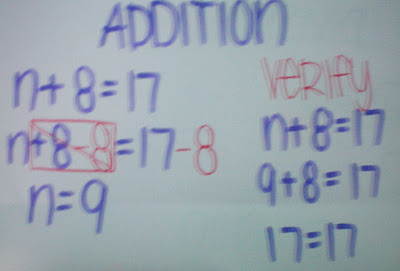### The Great Book of Algebra

Thursday, December 4, 2008
CHAPTER 1: Integer Poetry

Positive numbers are easy
Plus numbers are fun

Diamante- SUBTRACT

Subtract
Take away, decrease
Losing, removing, lessening
Add opposite like terms
Minusing, decreasing, diminishing
Reduce, minus
Negative

Free Verse- Partitive

Partitive
is sharing with what you have.
Partitive
is grouping numbers.
Partitive
is a way of dividing.
Partitive
is so easy!

Free Verse- Quotative

Quotative
is splitting into groups.
Quotative
is like normal dividing.
Quotative
is one of the three ways to divide.
Quotative
is very simple!

Free Verse- Ron's Rule

Ron's rule is a very simple rule
you can do it nothing to it
just remember the same sign's
are always positive and different
sign's are always negative.

CHAPTER 2: Combining like terms and Distributive Property

SCRIPT:
Kayla: Hey Shawn! How are you?
Shawn: Hey! I'm fine, and you?
Kayla: I'm fine, but can you help me with my math homework?
Shawn: Sure! What do you need help on?
Kayla: Well, my class and I are learning combining like terms and distributive property.
Shawn: Ah... that's easy. You'll understand in a jiffy.
Kayla: Okay, the first question is n+3-5n+12. How do I solve this question?
Shawn: Well, what do you think the answer is? I think it's... -6n+15, am I right or am I wrong?
Kayla: Your answer is close. The answer is actually -4n+15. How did you get the answer?
Shawn: First of all, you should circle the variable so you don't get confused with the other terms. Second, group like terms. This means put integers with the same variable together and the other integers together finally, simplify.
Kayla: Oh.. how do you simplify?
Shawn: To simplify, all you have to do is combine like terms.
Kayla: That's it? That is so easy!
Shawn: Of course it is once you know what to do. Is there anything else I can help you with?
Kayla: Actually, yes.. the next question has brackets.. i know I'm suppose to multiply, but I don't know which one to multiply.
Shawn: Whats the question?
Kayla: 2+4(3n+8)
Shawn: This is going to be easy, just like the first question!
Kayla: Wait, can I try this question on my own first?
Shawn: Sure, but tell me how you got the answer after.
Kayla: Well I think the answer is 12n+10. First of all, I knew the rule of multiply integers. So, I multiplied the 4 with 3n since there was a bracket. That's how I got 12n. I didn't know what to do with the other two numbers, so I added them together.

Chapter 2: Combining Like Terms and Distribute Property
It didn't work. I have it done but my video wont upload here.

CHAPTER 3: One Step Equation Solving
Here are some Addition, Subtraction Multiplying, and DivisionEquations !

RULES:
I
-isolate
C-cancelling using the
O-opposite
B-balance
V
-verify
*What you do to one side, you have to do the samething to the other side!"*The first question is n+8= 17. As you can see, I've isolated n by using the opposite of +8 (which is obviously -8). What you do on one side, you have to do the same thing on the other side. So, I put -8 beside 17 to balance it out. You're left with n= 9. That is how you solve n. Now, you have to verify so you can get full marks.
SUBTRACTION:

The second questions is n-7=10. The rules are similiar to the additive equations. Isolate to get n by using the opposites, balance and verify. It's like adding, you need to isolate the variable by cancelling out using opposites. I've isolated n by using the opposite of -7 (which is obviously +7). Don't forget to balance it out by doing what you do to one side, so you do the same thing to the other side. Now, your left with n=17. After the question has been solved all you have to do is verify.

MULTIPLYING:The third question is 4n=-8. Again, the rules still apply to multiplication as well. When you're isolating n in a multiplication equation, you divide since you need to cancel them out. You do the same thing to the other side. Now , you have to figure out what -8/4 is in order to get n. Your left with n=-2. Now that your done solving the question, you have to verify.

DIVISION:

Finally, the last question is n/4=3. After that, you do the same thing again as the rest of the questions we did. You have to multiply to isolate n when you're doing a division equation. Do the same thing to the other side. Figure out what 3(4) is. Which leaves you with n= 12. After solving the question you Verify. Now you know how to do one step equations!

CHAPTER 4: Algetile Video

During math class , we were told to make a video about a algebra equations using algetiles. We had to work on it at lunch, because we didn't get to finish it during class. So, sorry for the background noise. Props to: Hanbit, Maeddah, and Tracy.